Energy Profiles An energy profile shows the energy changes taking place during a chemical reaction. It provides some very useful information relating to the reaction. The point of any energy profile is to show the relative energies (enthalpies) of reactants and products. Energy profiles have meaningful y-axis but not a meaningful x-axis. It should be stressed that the x-axis is not 'time' and can not be used to derive information of the rate of reaction. Consider the reaction between nitrogen dioxide and carbon monoxide, shown below. NO2(g) + CO(g) => NO(g) + CO2(g) The energy profile of this reaction is shown on the right. It reveals that there is an energy difference between the reactants and the products of 225 kJ for every mol of NO that reacts with one mol of CO. It shows the total chemical energy of the products to be lower than the total chemical energy of the reactants. We therefore right the equation as NO2(g) + CO(g) => NO(g) + CO2(g) ΔH = - 225 kJ/mol. The negative sign showing that energy is given out. This is an exothermic reaction. It is also shown that 116 kJ/mol of energy is needed to get the reaction started. This is known as the activation energy. Initially the reactants have little energy to react and the collisions that do occur have no consequence. When enough energy is added the collisions have greater energy to break bonds and form an activated complex. This is a very unstable intermediate that has an extremely short life-span. The activated complex breaks apart into product molecules releasing 225 kJ of energy.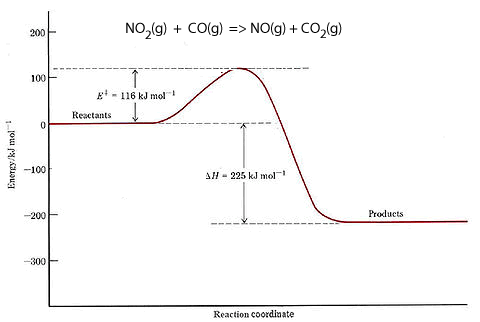We can also see from the energy profile that the reverse reaction NO(g) + CO2(g) => NO2(g) + CO(g) ΔH = +225 kJ/mol. will require 341 kJ/mol of activation energy and absorb 225 kJ/mol. It is worth noting that the activation energy determines the rate of the reaction. The greater the activation energy the slower the reaction. So the reverse reaction, for the exothermic reaction shown on the right, has a greater activation energy than the forward reaction. Hence the reverse reaction proceeds at a slower rate than the forward reaction. There comes a time when the amount of reactants is so low that the forward reaction slows down to equal the backward reaction. At this point equilibrium is said to have been achieved.Consider the two energy profiles shown on the right. Which reaction proceeds forward at the slowest rate? Explain with reference to average kinetic energy. Which reaction proceeds backwards at the fastest rate? What is the activation energy of the forward reaction for reaction B ? 290 kJ 200 kJ 590 kJ What is the activation energy of the backward reaction for reaction B ? 290 kJ 200 kJ 490 kJ What is the ΔH of reaction A? -290 kJ +200 kJ -160 kJ +160 kJ Is heat produced when reaction A proceeds in the forward direction? Yes No Is heat produced when reaction B proceeds in the backward direction? Yes No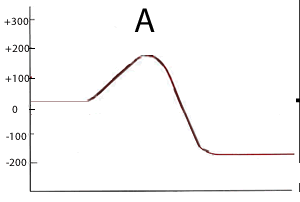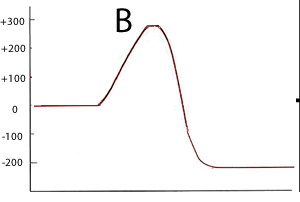Lets take the formation of hydrogen chloride from hydrogen and chlorine gases. H2(g) + Cl2(g) ==> 2HCl(g) Now the bond energies in kJ/mol for each bond are: H-H = 436; Cl-Cl = 242; H-Cl = 431. To break the bonds of one mol of each reactant molecule we need 436 + 242 = 678 kJ to be provided. We have 2 mol of product (HCl) of which each mol of has 431 kJ of bond energy. So the total bond energy of the products is 862. The net difference between them = 862-678 = 184 kJ given out. H2(g) + Cl2(g) ==> 2HCl(g) ΔH = -184 kJ/mol This tells us that when two mol of HCl are formed 184 kJ of energy is given out. The reaction is exothermic since more energy is given out than taken in. Why is the bond energy of the products and the activation energy the same? is this always the case? Explain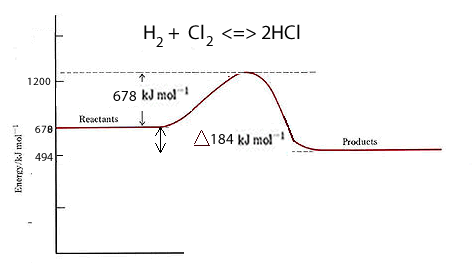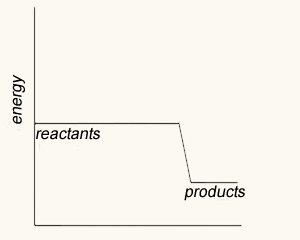Before attempting this exercise read this article on activation energy. Match the energy profile with the appropriate statement. a moderately exothermic reaction with no activation energy a highly exothermic reaction between gaseous ions a highly exothermic reaction with a large activation energy a moderately endothermic reaction that occurs at a relatively slow rate a moderately endothermic reaction that occurs at a relatively fast rate an exothermic reaction with a moderate activation energy.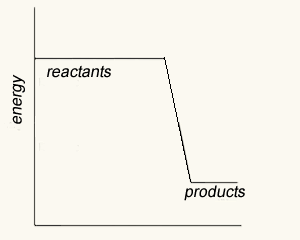a moderately exothermic reaction with no activation energy a highly exothermic reaction between gaseous ions a highly exothermic react in with a large activation energy a moderately endothermic reaction that occurs at a relatively slow rate a moderately endothermic reaction that occurs at a relatively fast rate an exothermic reaction with a moderate activation energy.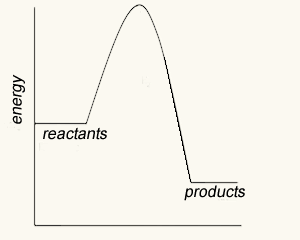a moderately exothermic reaction with no activation energy a highly exothermic reaction between gaseous ions a highly exothermic reaction with a large activation energy a moderately endothermic reaction that occurs at a relatively slow rate a moderately endothermic reaction that occurs at a relatively fast rate an exothermic reaction with a moderate activation energy.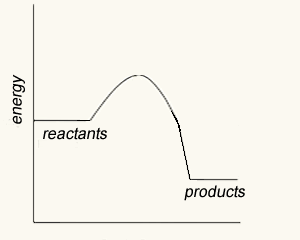a moderately exothermic reaction with no activation energy a highly exothermic reaction between gaseous ions a highly exothermic reaction with a large activation energy a moderately endothermic reaction that occurs at a relatively slow rate a moderately endothermic reaction that occurs at a relatively fast rate an exothermic reaction with a moderate activation energy.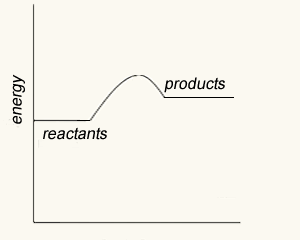a moderately exothermic reaction with no activation energy a highly exothermic reaction between gaseous ions a highly exothermic reaction with a large activation energy a moderately endothermic reaction that occurs at a relatively slow rate a moderately endothermic reaction that occurs at a relatively fast rate an exothermic reaction with a moderate activation energy.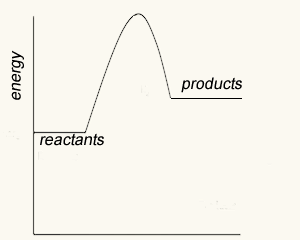a moderately exothermic reaction with no activation energy a highly exothermic reaction between gaseous ions a highly exothermic reaction with a large activation energy a moderately endothermic reaction that occurs at a relatively slow rate a moderately endothermic reaction that occurs at a relatively fast rate an exothermic reaction with a moderate activation energy.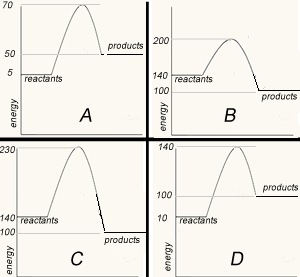The image on the right shows four energy profile diagrams of 4 reactions . a) If energy profile A represents the reaction , 2a + 3b => 4C + 4 D,which profile represents the reaction a + 3/2 b => 2C + 2D? A B C D b) Consider the reactions represented by the energy profiles C and D. These reactions occcur under similar conditions. Which reaction occurs at the fastest rate? A B C D Explain c) Which of the profiles show a catalysed reaction? A B C D Explain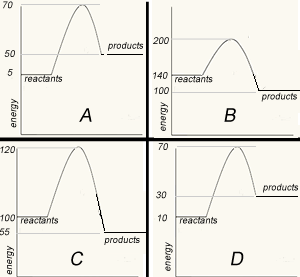The image on the left shows four energy profile diagrams of 4 reactions . If energy profile A represents the reaction a + b => C + F, which profile represents the reaction C + F => a + b? A B C D Explain What is the ΔH of the reaction represented by profile A? Solutions